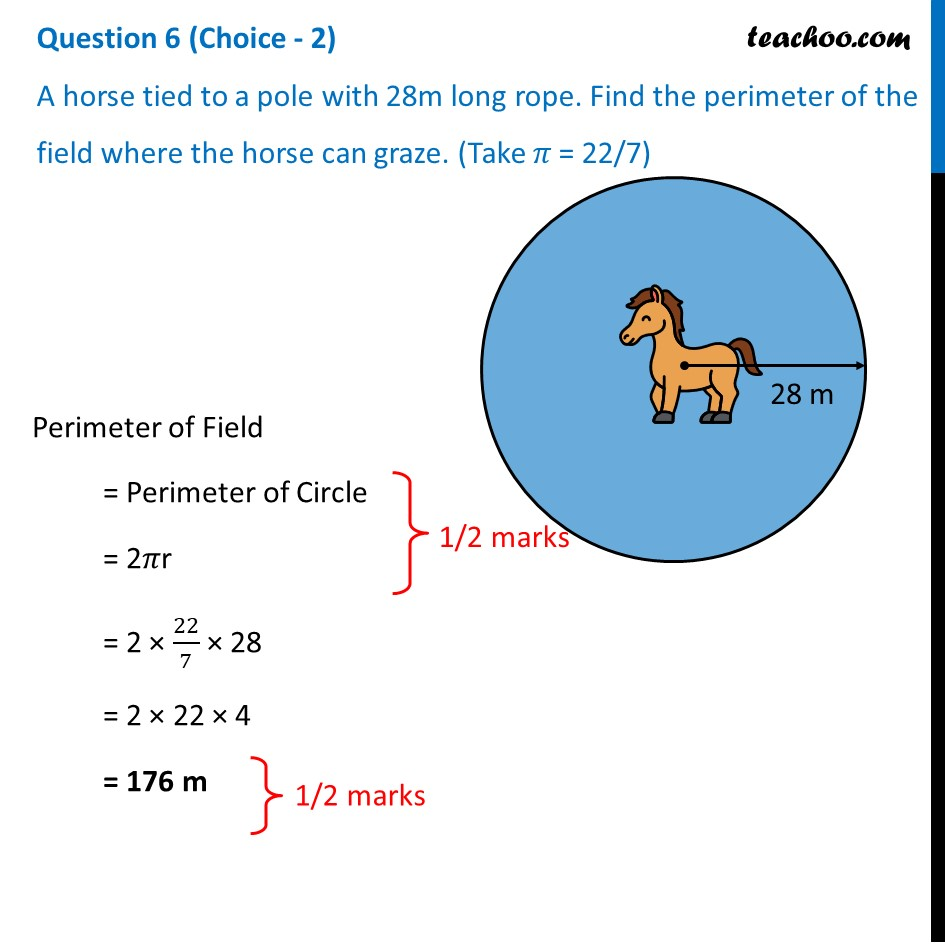A horse tied to a pole with 28m long rope. Find the perimeter of the field where the horse can graze. (Take 𝜋 = 22/7)1. Class 10
2. Solutions of Sample Papers for Class 10 Boards
3. CBSE Class 10 Sample Paper for 2021 Boards - Maths Basic

Transcript

Question 6 (Choice - 2) A horse tied to a pole with 28m long rope. Find the perimeter of the field where the horse can graze. (Take 𝜋 = 22/7) Perimeter of Field = Perimeter of Circle = 2𝜋r = 2 × 22/7 × 28 = 2 × 22 × 4 = 176 m

CBSE Class 10 Sample Paper for 2021 Boards - Maths Basic

Class 10
Solutions of Sample Papers for Class 10 Boards